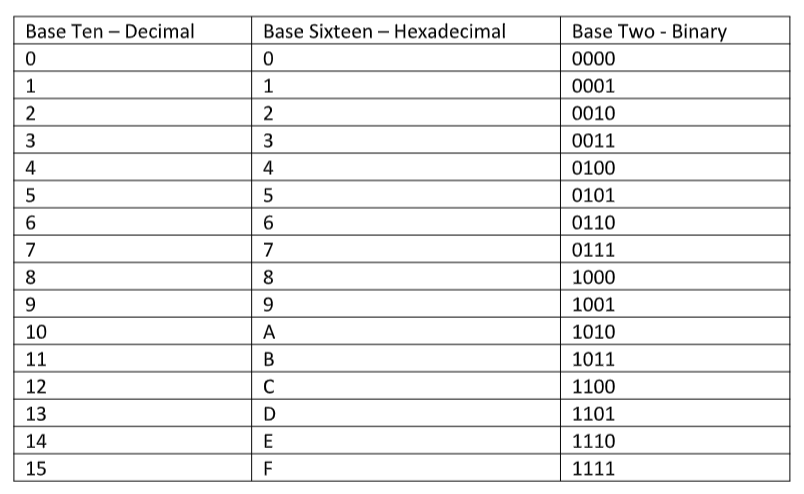# Hexadecimal and Binary Numbering Systems

• June 29, 2020
• Bryan Hennessy

I have not been able to find a good hexadecimal explanation site, so here’s an overview in my own words, with a few links for you to explore.

Understanding hexadecimal (hex) and binary is all about understanding numbering systems, or number bases. What we use every day is base ten, hexadecimal is base sixteen and binary is base two.

### Base ten is decimal

In base ten, we have ten digits that represent a number; zero through nine, or 0, 1, 2, 3, 4, 5, 6, 7, 8, and 9. When we get to 9, we shift left and start over at 10, 11, 12, 13,……19. We then go to 20.

Large decimal numbers are shown in sets of three with a comma between sets; 123,456,789.

### Base sixteen is hexadecimal, or 'hex'

In base sixteen, we have sixteen digits that represent a number; zero through F, or 0, 1, 2, 3, 4, 5, 6, 7, 8, 9, A, B, C, D, E, and F. When we get to F, we shift left and start over at 10, 11, 12, 13, … 1F. We then go to 20.

Large hexadecimal numbers are shown in sets of two with a space between sets; 78 9A BC DE. A set of two hex characters is called a byte.

### Base two is binary

In base two, two digits represent a number; zero and one, or 0 and 1. When we get to 1, we shift left and start over at 10, 11, 100, 101, 110, … 1111. We then go to 0001 0000.

Large binary numbers are shown in sets of four with a space between sets, and zeros are used to make all sets of four; ‘0001 0101 1101 1111’.

### Binary vs hex

There is a relationship between binary and hex. One hex character represents a block of four binary characters. See the table below:A binary character is called a bit. Computers and digital communications work in binary.

Eight bits make up a byte, and a byte is usually represented by two hex characters, like 3E or B8.